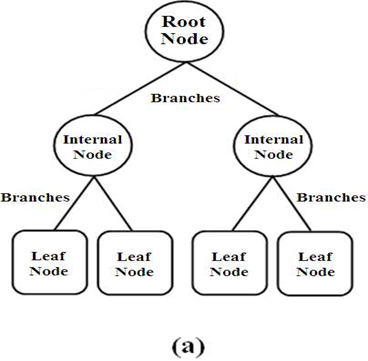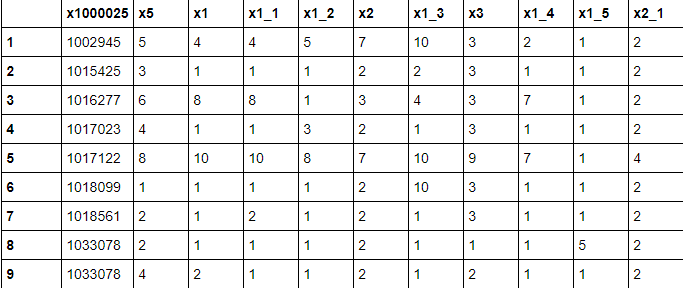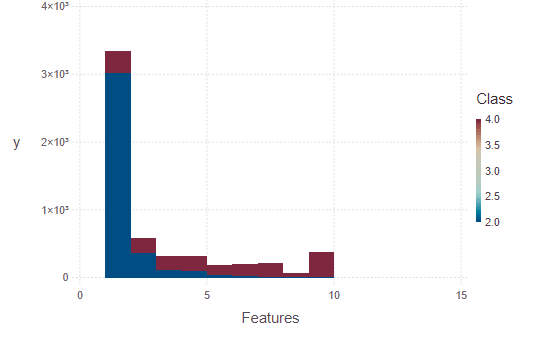# Decision Tree Classifiers in Julia

• Last Updated : 01 Nov, 2020

In statistics in Julia, classification is the problem of identifying to which of a set of categories (sub-populations) a new observation belongs, on the basis of a training set of data containing observations (or instances) whose category membership is known.

In the terminology of machine learning, classification is considered an instance of supervised learning, i.e., learning where a training set of correctly identified observations is available.

Some of the classification techniques which we have are:

1. Linear Classifiers: Logistic Regression, Naive Bayes Classifier
2. Nearest Neighbor
3. Support Vector Machines
4. Decision Trees
5. Boosted Trees
6. Random Forest
7. Neural Networks

### Decision tree classifiers

A Decision Tree is a simple representation of classifying examples. It is a Supervised Machine Learning where the data is continuously split according to a certain parameter.

Decision trees are commonly used in operations research and operations management. If in practice, decisions have to be taken online with no recall under incomplete knowledge, a decision tree should be paralleled by a probability model as the best choice model or online selection model algorithm. Another use of decision trees is as a descriptive means for calculating conditional probabilities.

A decision tree has mainly three components:

1. Root Nodes: It represents the entire population or sample and this further gets divided into two or more homogeneous sets.
2. Edges/Branch: Represents a decision rule and connect to the next node.
3. Leaf nodes: Leaf nodes are the nodes of the tree that have no additional nodes coming off them. They don’t split the data any further### Implementation of Decision Tree Classifiers in Julia

Decision Tree is a flow chart like structure

• use axis-aligned linear decision boundaries to partition or bisect data
• Divide and conquer approach

Packages and Requirements

## Julia

 `# using the packages``using DataFrames``using DecisionTree`` ` `# Loading the Data``# https://archive.ics.uci.edu/ml/machine-learning-databases/breast-cancer-wisconsin/``df ``=` `readtable(``"breastc.csv"``)`

Output:## Julia

 `# using gadly package``using Gadfly`` ` `plot(df, x ``=` `Xfeatures,``     ``y ``=` `Ylabel, Geom.histogram, ``     ``color ``=` `:Class, ``     ``Guide.xlabel(``"Features"``))`

Output:My Personal Notes arrow_drop_up